# AP Chemistry : System Equilibrium

## Example Questions

### Example Question #1 : System Equilibrium

What is occuring when a mixture reaches a dynamic equilibrium state?

Neither reaction is occurring; the mixture is completely static

Only the reverse reaction is occurring

Only the forward reaction is occurring

The forward and reverse reactions are occurring at the same rate

The forward and reverse reactions are occurring at the same rate

Explanation:

Dynamic equilibrium means that the forward and reverse reactions are occurring at the same rate so that there is no net change in the concentrations.

### Example Question #41 : Chemical Equilibrium

Which of the following accurately describes what occurs in reversible reactions?

The reaction can proceed in both the forward and reverse directions, and these reactions do not usually go to completion.

none of the above

The reaction can proceed in both the forward and reverse directions, and these reactions always go to completion.

The reaction can proceed in the forward direction to completion.

The reaction can proceed in both the forward and reverse directions, and these reactions do not usually go to completion.

Explanation:

The definition of reversible reactions is that they are indeed reversible; in other words, they can proceed in the forward and reverse directions. Since the products can react as well to reform the reactants, these reactions usually do not proceed to completion and instead exist in a dynamic state.

### Example Question #3 : System Equilibrium

Consider the following reaction: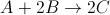The equilibrium constant,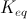, is 0.367.

In a reaction flask, you have a solution containing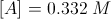,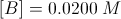, and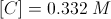. Will the reaction proceed forward (to the right), or backwards (to the left)?

The reaction will proceed to the right

The reaction will proceed both to the right and two the left, but at equal rates

The reaction will proceed to the left

The reaction will not proceed

The reaction will proceed to the left

Explanation:

For the reaction:The expression for the equilibrium constant is: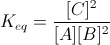The reaction quotient is: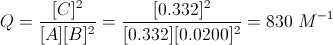The reaction quotient is much larger than the equilibrium constant, so the reaction will proceed to the left.

### Example Question #41 : Chemical Equilibrium

Which of the following is not always true for the given arbitrary reaction in equilibrium?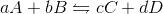The molar concentrations of reactants and products are equal

The forward and reverse reaction rates are equal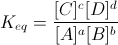Each choice is always true for this equilibrium reaction

The molar concentrations of reactants and products are equal

Explanation:

The forward and reverse reaction rates are equal for reactions in equilibrium, however, the molar concentration of the reactants and products are usually not equal themselves. One can calculate the equilibrium constant using the equation below.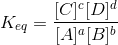### Example Question #5 : System Equilibrium

Determine the equilibrium expression for the following reaction: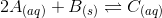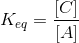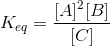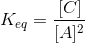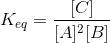Explanation:

Equilibrium expressions are a ratio of the concentration of products raised to the stoichiometric coefficient, divided by reactants raised to the stoichiometric coefficient. Solids are omitted from this expression.

For the generic example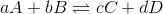, the equilibrium expression is.

For our given reaction, we can find the equilibrium expression in a similar manner. Do not forget to omit the solid component of the reaction!